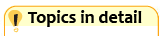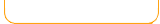Use pictures to learn addition Add using number lines. Shoot the fruit that solves the addition problem. Add with animated multiple choice fun. Bubble Fun Math Basics Bubble Fun Math Practice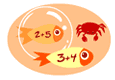Put bubbles around the sea creatures that solves the addition problem. (Number ranges)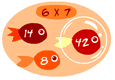Put bubbles around the sea creatures that solves the addition problem. (Practice individual numbers) Math Man Addition Arcade math game - add correctly in this pac-man like game. Racing game - drive into the correct answers.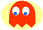1 to 50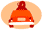doublesCatch the stars and practice addition.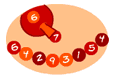Match and shoot balls to form pairs that add to the given number.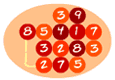Find pairs of numbers that add up to the target number.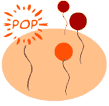Pop balloon while ordering addition sums. Addition with missing numbers Matching Addition Solve for the missing number. A matching game.Match addition problems. Math Madness Addition Match tiles with multiplication and solve the puzzle. Addition arcade game. Bus Driver's Math Fruit Shoot Skill BuildersAdd to find out if you have enough money for the bus.Shoot the fruit that solves the addition problem. Add by numbers, doubles and near doubles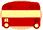Level 3